# How to Statistic Pass 4 Out of 5 Rounds in a Competition by Excel Functions

Suppose you are counting the numbers of participants who are the winners in a competition. And the passing line is provided as pass 4 out of 5 rounds of games. You need to know if participant pass the game or not firstly. If you are familiar with the functions in Excel and can use them expertly, you can work out this issue easily. By the way, if you are confused of choosing and applying which function among all Excel functions, this article will help you to solve your problem.

In this article, through explaining the example below, we will introduce you to apply IF and COUNTIF functions together to return win or lose for each participant. You can get the basic knowledge and usage of these two functions in this article.

## EXAMPLE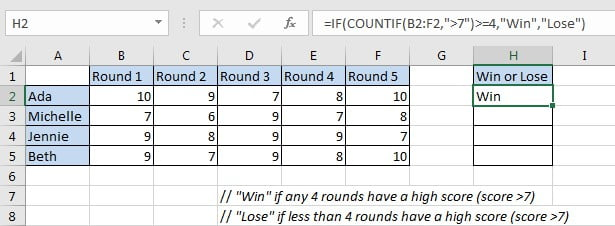In this case, we want to know if participants win or lose the game. “Win” is returned if participant passed four rounds of games with a high score; otherwise “Failed” is returned.

In this article, to approach our goal, we applied IF function with COUNTIF function inside.

## SOLUTION

In this instance, the final output in “Win of Lose” column is “Win” or “False”, the result depends on if participants passed any four rounds of games with high a high score. To output either A or B based on one condition, we can apply IF function here. This function is frequently used in the situations like ‘to return A or B by a given precondition”.

To count number of rounds with a high score, we can select COUNTIF function as it can count with one condition properly.

Besides, COUNTIF has some related functions like COUNT and COUNTIFS. COUNT function returns number of cells which contain a numeric value; COUNTIF is COUNT+IF, it returns number of cells with one condition or criterion; if there are multiple criteria, we can apply COUNTIFS.

## FORMULA with IF & COUNTIF FUNCTION

In H2, we input the formula =IF(COUNTIF(B2:F2,”>7″)>=4,”Win”,”Lose”). After typing, press Enter, verify that “Win” is displayed in H2. As Ada has a high score in round-1, round-2, round-4 and round-5, she passed the game with 4-rounds high scores, so “Win” is returned for her.Drag down handle to fill other cells with the same formula in “Win or Lose” column.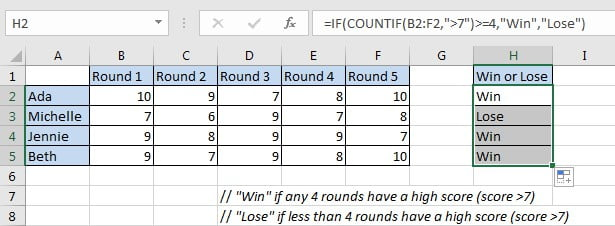Michelle lose the game as she only passes the round-3 and round-5 with a high score.

## FUNCTION INTRODUCTION

In this instance, returning “Win” or “False” depends on the result of whether participants passed four rounds of games with a high score, the final output “Win” of “False” comes from IF function. To determine if participants passed the game with 4 or more high scores, we applied COUNTIF function here.

a. COUNTIF returns the number of cells which contain a number and the number meets one condition or criterion. The condition or criterion is determined by our input in “criteria” argument.

Syntax:

`=COUNTIF(range, criteria)`

Example.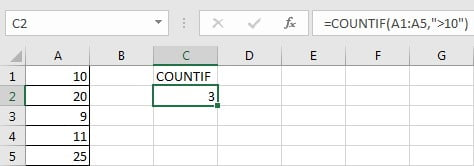b. IF function returns “true value” or “false value” based on the result of provided logical test. It is one of the most popular function in Excel.

Syntax:

`=IF(logical_test,[value_if_true],[value_if_false])`

Example.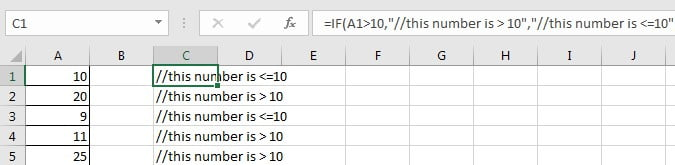## FORMULA EXPLANATION

`=IF(COUNTIF(B2:F2,">7")>=4,"Win","Lose")`

// logical test is “COUNTIF(B2:F2,”>7″)>=4”

// value of true is “Win”

// value of false is “Lose”

c. COUNTIF(B2:F2,”>7″) is used for counting the number of cells from range B2:F2 with the condition number in cell should be “greater than 7”. In this case, this expression is used for counting the rounds number with a high score 8, 9 or 10 for Ada. Expand values in B2:F2, we get below expression:

COUNTIF({10,9,7,8,10},”>7″) // returns 4

d. COUNTIF returned value “4” comes to the logical expression “COUNTIF(B2:F2,”>7″)>=4” inside IF function. In this logical test “4>=4” is true, so the value of true “Win” is returned by this formula finally.

=IF(4>=4,”Win”,”Lose”) // returns “Win”

## EXPAND

If we add two new conditions that round-1 and round-2 must pass with high score, current formula cannot satisfy our demand. Now, the passing line for “Win” is updated to must meet below three conditions:

1. The initial condition “COUNTIF(B2:F2,”>7″)>=4”.
2. Round-1 has a high score “B2>7”.
3. Round-2 has a high score “C2>7”.

Here, we can apply another function AND to concentrate the three conditions in one expression. AND function can return a “TRUE” if all conditions are met.

Update formula to =IF(AND(COUNTIFS(B2:F2,”>7″)>=4,B2>7,C2>7),”Win”,”Lose”).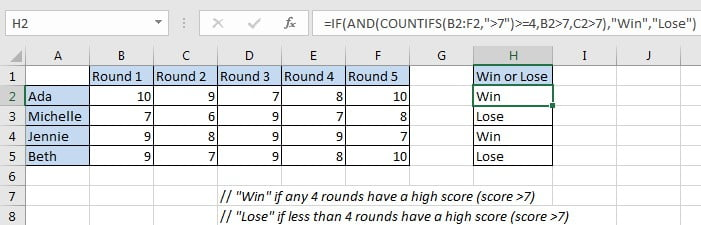### Related Functions

• Excel COUNTIF function
The Excel COUNTIF function will count the number of cells in a range that meet a given criteria. This function can be used to count the different kinds of cells with number, date, text values, blank, non-blanks, or containing specific characters.etc.= COUNTIF (range, criteria)…
• Excel COUNTIFS function
The Excel COUNTIFS function returns the count of cells in a range that meet one or more criteria. The syntax of the COUNTIFS function is as below:= COUNTIFS(criteria_range1, criteria1, [criteria_range2, criteria2]…)…
• Excel IF function
The Excel IF function perform a logical test to return one value if the condition is TRUE and return another value if the condition is FALSE. The IF function is a build-in function in Microsoft Excel and it is categorized as a Logical Function.The syntax of the IF function is as below:= IF (condition, [true_value], [false_value])….
• Excel AND function
The Excel AND function returns TRUE if all of arguments are TRUE, and it returns FALSE if any of arguments are FALSE.The syntax of the AND function is as below:= AND (condition1,[condition2],…)…